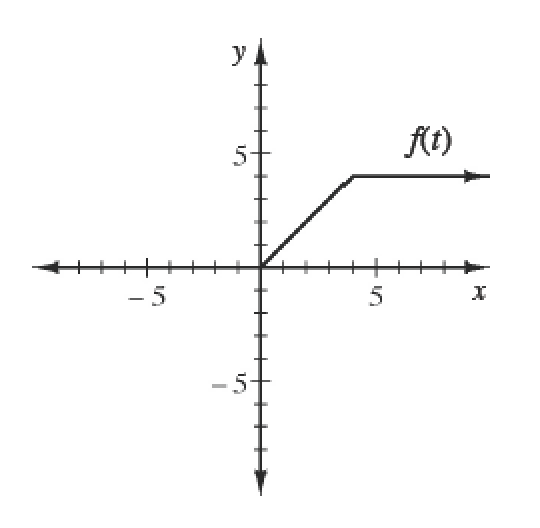### Home > APCALC > Chapter 4 > Lesson 4.2.3 > Problem4-77

4-77.
1. If $f$ is an odd function and $g ( x ) = \int _ { 0 } ^ { x } f ( t ) d t$, evaluate:

Sketch the graph of the odd function, $f(t)$.

1. $g(–3)$

2. $2 · g(–7)$

3. $g(9) + g(–9)$

2. If $f$ is an even function and $g ( x ) = \int _ { 0 } ^ { x } f ( t ) d t$, evaluate:

Sketch the graph of the even function, $f\left(t\right)$.

1. $g(–3)$

2. $2 · g(–7)$

3. $g(9) + g(–9)$

Use the eTool below to help solve this problem.
Click the link at right for the full version of the eTool: Calc 4-77 HW eTool